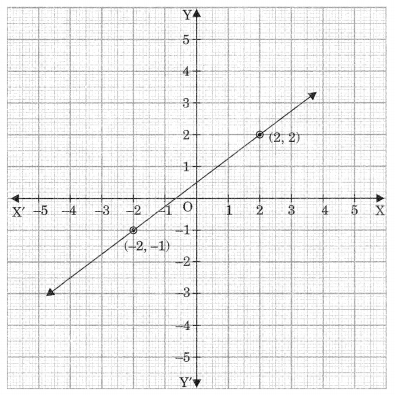Check the below Online Education NCERT MCQ Questions for Class 9 Maths Chapter 4 Linear Equations for Two Variables with Answers Pdf free download. MCQ Questions for Class 9 Maths with Answers were prepared based on the latest exam pattern. We have provided Linear Equations for Two Variables Class 10 Maths MCQs Questions with Answers to help students understand the concept very well. https://ncertmcq.com/mcq-questions-for-class-9-maths-with-answers/

Students can also refer to NCERT Solutions for Class 9 Maths Chapter 4 Linear Equations for Two Variables for better exam preparation and score more marks.

## Online Education Linear Equations for Two Variables Class 9 MCQs Questions with Answers

Linear Equations In Two Variables Class 9 MCQ Question 1.
The value of y at x = -1 in the equation 5y = 2 is
(a) $$\frac{5}{2}$$
(b) $$\frac{2}{5}$$
(c) 10
(d) 0

Answer: (b) $$\frac{2}{5}$$

Class 9 Maths Chapter 4 MCQ Question 2.
Equation of a line which is 5 units distance above the x-axis is
(a) x = 5
(b) x + 5 = y
(c) y – 5
(d) x – y = 0

This calculator will solve the system of linear equations calculator of any kind.

Linear Equations In Two Variables MCQ Class 9 Question 3.
x = 3 and y = -2 is a solution of the equation 4px – 3y = 12, then the value of p is
(a) 0
(b) $$\frac{1}{2}$$
(c) 2
(d) 3

Answer: (b) $$\frac{1}{2}$$

Ch 4 Maths Class 9 MCQ Question 4.
Which of the following is the equation of a line parallel to y-axis?
(a) y = 0
(b) x + y = z
(c) y = x
(d) x = a

Class 9 Linear Equations In Two Variables MCQ Question 5.
Any point on the line y = 3x is of the form
(a) (a, 3a)
(b) (3a, a)
(c) (a, $$\frac{a}{3}$$)
(d) ($$\frac{a}{3}$$, -a)

Question 6.
To which equation does the graph represent?(a) 3x – 7y = 10
(b) y – 2x = 3
(c) 8y – 6x = 4
(d) 5x +$$\frac{35}{2}$$y = 25

Answer: (c) 8y – 6x = 4

Question 7.
Any point of the form (a, – a) always lie on the graph of the equation
(a) x = -a
(b) y = a
(c) y = x
(d) x + y = 0

Answer: (d) x + y = 0

Question 8.
The graph of the equation 2x + 3y = 6 cuts the x-axis at the point
(a) (0, 3)
(b) (3, 0)
(c) (2, 0)
(d) (0, 2)

Question 9.
Graph of linear equation ax + by + c = 0, a * 0, 6*0 cuts x-axis and y-axis respectively at the points.
(a) ($$\frac{-c}{a}$$, 0), (0, $$\frac{-c}{b}$$)
(b) (0, $$\frac{-c}{b}$$, 0), ($$\frac{-c}{a}$$, 0)
(c) (-c, 0) (0, -c)
(d) (x, 0) (y, 0)

Answer: (a) ($$\frac{-c}{a}$$, 0), (0, $$\frac{-c}{b}$$)

Question 10.
Which of the following ordered pairs is a solution of the equation x – 2y – 6?
(a) (2, 4)
(b) (0, 3)
(c) (-4, 1)
(d) (4, -1)

Question 11.
How many linear equation in x and y can be satisfied by x = 1 and y = 2?
(a) only one
(b) two
(c) infinitely many
(d) three

Question 12.
Solution of linear equation 2x + 0.y + 9 = 0 is
(a) ($$\frac{9}{2}$$, m)
(b) (n, $$\frac{-9}{2}$$)
(c) (0, $$\frac{-9}{2}$$)
(d) ($$\frac{-9}{2}$$, 0)

Answer: (d) ($$\frac{-9}{2}$$, 0)

Question 13.
If (3, 2) is the solution 3x – ky = 5, then k equals of the equation
(a) 2
(b) 4
(c) 3
(d) $$\frac{1}{2}$$

Question 14.
Cost of book (x) exceeds twice the cost of pen (y) by Rs 10. This statement can be expressed as linear equation.
(a) x – 2y – 10 = 0
(b) 2x – y – 10 = 0
(c) 2x + y – 10 = 0
(d) x – 2y + 10 = 0

Answer: (a) x – 2y – 10 = 0

Question 15.
If x represents the age of father and y represents the present age of the son, then the statement for ‘present age of father is 5 more than 6 times the age of the son’ in terms of mathematical equation is
(a) 6x + y = 5
(b) x = 6y + 5
(c) x + 6y = 5
(d) x – 6 = 5

Answer: (b) x = 6y + 5

Question 16.
Equation of a line passing through origin is
(a) x + y = 1
(b) x = 2y – 4
(c) x + y = 0
(d) y = x – 1

Answer: (c) x + y = 0

Question 17.
The condition that the equation ax + by + c = 0 represents a linear equation in two variables is
(a) a ≠ 0, b = 0
(b) b ≠ 0, a = 0
(c) a = 0, b = 0
(d) a ≠ 0, b ≠ 0

Answer: (d) a ≠ 0, b ≠ 0

Question 18.
The maximum number of points that lie on the graph of a linear equation in two variables is.
(a) two
(b) definite
(c) infinitely many
(d) three

Question 19.
Straight line passing through the points (-1, 1), (0, 0) and (1, -1) has equation
(a) y – x
(b) x + y = 0
(c) y = 2x
(d) 2 + 3y = 7x